Home Help Search Login Register
 2021-12-01, 02:32:31News: Forum TIP:The SHOUT BOX deletes messages after 3 hours. It is NOT meant to have lengthy conversations in. Use the Chat feature instead. 1 Hour 1 Day 1 Week 1 Month Forever

Pages: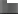Author Topic: Inductance measurement  (Read 4899 times) Topic Options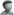exnihiloest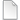Inductance measurement « on: 2012-11-10, 11:29:35 » Group: Guest Here is an extremely simple method from Ronald Dekker, recently reworked by JL Naudin, to measure inductances. It works well: connect directly a signal generator to an oscilloscope. The generator must have a 50Ω output impedance. Note the voltage. connect in parallel the unknown inductance. adjust the frequency F so that the voltage to be half the previous voltage.The inductance is: L = 4.57/F  (Henry).From jnaudin.free.fr: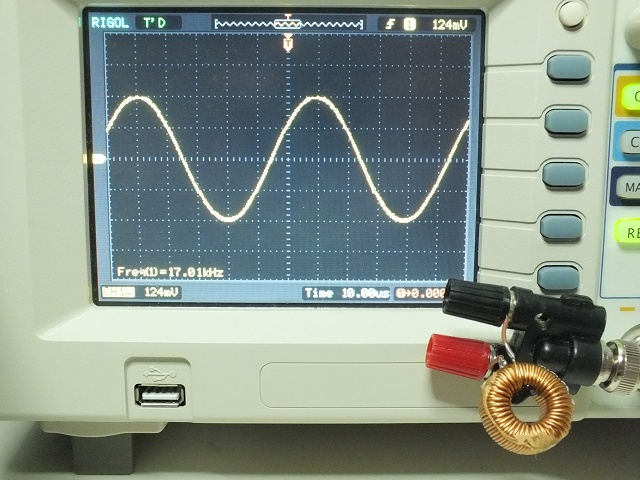forestRe: Inductance measurement « Reply #1 on: 2012-11-10, 20:51:55 » Sr. Member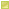Posts: 340 I cannot use that because of missing signal generator (probably sinewave is needed, right ?) . Can you test if it's usable to test inductance of iron core ordinary transformers like E+I core based ? I have inductance meter but value if floating and not stable ( I suppose because of saturation of core ?).exnihiloestRe: Inductance measurement « Reply #2 on: 2012-11-10, 21:42:17 » Group: Guest @forestYour are right to specify that a sinewave is needed.An inductance is an inductance whatever the way it is made. But if it is non linear and depends on parameters, as an inductance with an iron core is when near  saturation, the method will work while the signal is in a range where the inductance can be considered constant, for example the primary coil of a mains transformer tested with a 1v pp signal. The measured inductance will give the inductance for this range only. If a cc current was superposed and saturated the core, another correct value would be measured for this new condition, always providing that the voltage range from the signal generator is low enough to not modify the inductance.The method is based on equation E = - L*(dI/dt). If L is not constant E = -d(LI)/dt = - L*(dI/dt) - I*dL/dt: the method fails if I*dL/dt is not negligible in comparison with L*di/dt, and so "I" must be low and consequently the voltage from the generator also, so that I*dL/dt~0.
Pages: